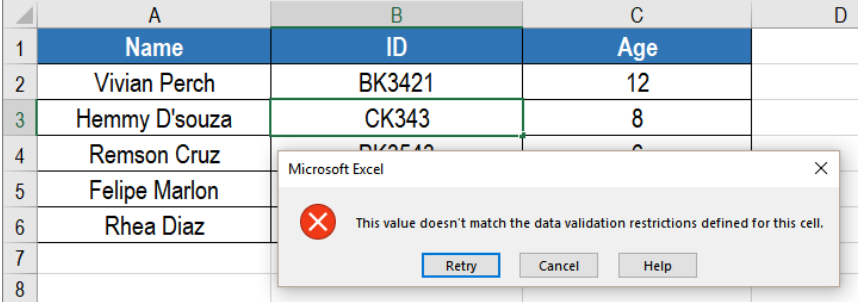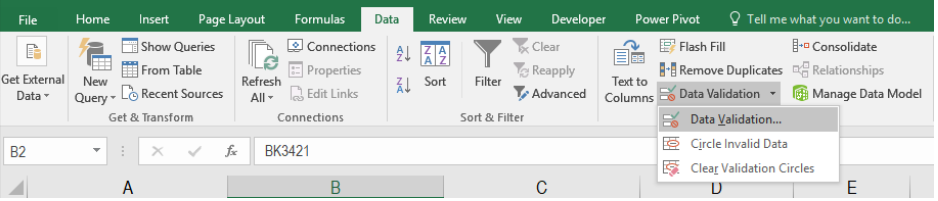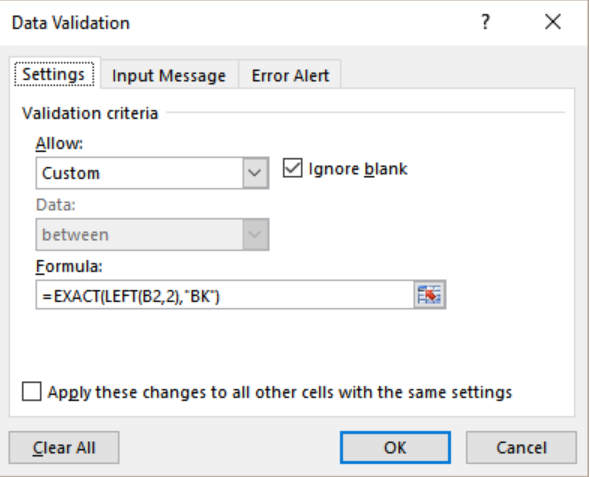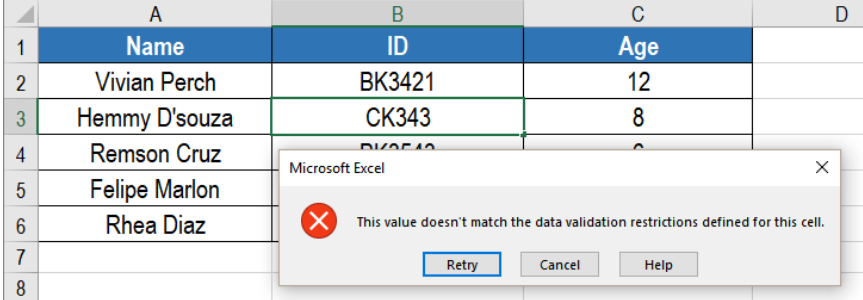Get instant live expert help with Excel or Google Sheets“My Excelchat expert helped me in less than 20 minutes, saving me what would have been 5 hours of work!”

#### Post your problem and you’ll get Expert help in seconds.

Your message must be at least 40 characters
Our professional Expert are available now. Your privacy is guaranteed.

# Learn What Data Validation Must Begin with in Excel

Data validation is a powerful feature in Excel. We can use it to control how a string begins with. Using the EXACT and LEFT function with data validation, we can control how a value begins with. In this tutorial, we will learn how to validate the start of a value in Excel.Figure 1. Example of how Data Validation Must Begin with in Excel

## Generic Formula

`=EXACT(LEFT(value,k),begin_with)`

## How the Formula Works

We can control how a cell value begins with data validation. The formula uses the EXACT and LEFT functions. The LEFT function extract the first k characters from the value.

Then, the EXACT function compares this text to the text provided into the formula. EXACT uses a case-sensitive approach in the comparison. EXACT returns TRUE when the two text strings match. If the match fails, Otherwise the validation fails and EXACT returns FALSE.

## Setting up Data

The following contains an student information database. We will fill these up. Column A, B and C will have the name, id and classes.

To restrict the ids to begin with “Bk”, we need to:

• Go to cell B2. Select cells B2 to B6 by clicking on B2 and dragging it till B6 with our mouse.
• Go to Data> Data Validation. We need to click the settings tab.Figure 2. Setting the Data Validation Rule

• Next, we have to click on the drop down on the Allow box.
• Here, we select Custom and in the formula box write the formula =EXACT(LEFT(B2,2),”Bk”).Figure 3. Applying the Formula to Data Validation

• Finally, we need to click OK.

This will only allow texts that start with “Bk” in column B. .Figure 4. Error Message with Data Validation

Excel will show an error if we start the ids with anything other than “BK”.

Most of the time, the problem you will need to solve will be more complex than a simple application of a formula or function. If you want to save hours of research and frustration, try our live Excelchat service! Our Excel Experts are available 24/7 to answer any Excel question you may have. We guarantee a connection within 30 seconds and a customized solution within 20 minutes.

### Did this post not answer your question? Get a solution from connecting with the expert.Another blog reader asked this question today on Excelchat:
Solution examplesUse the Vlookup Function to complete the "employee" column of table 2. Use "job Id" from table 2 as your lookup_value(s) and table 1 as your reference.
Solved by C. H. in 16 minsIf a cell in another sheet is populated I need a vlookup done. If the cell is not populated I need the cell to return blank.
Solved by T. D. in 60 minsI am trying to make a chart that turns a week range red if nothing is entered in the range. If something is entered then I would like it to turn green. Please Help
Solved by E. U. in 43 minsI need a check box to show/hide an answer of an if function
Solved by Z. U. in 23 minsI need a formula to compare the data in two columns and then export the mismatched data in the 3rd column
Solved by S. Q. in 20 mins## Subscribe to Excelchat.coAnother blog reader asked this question today on Excelchat: